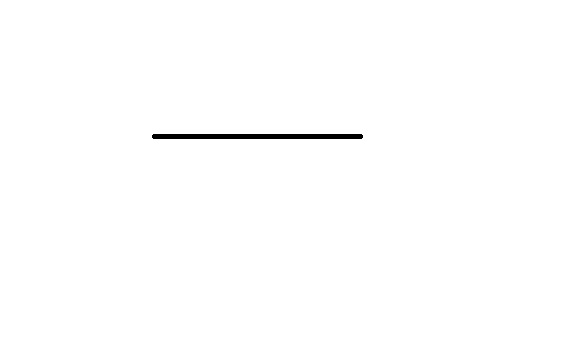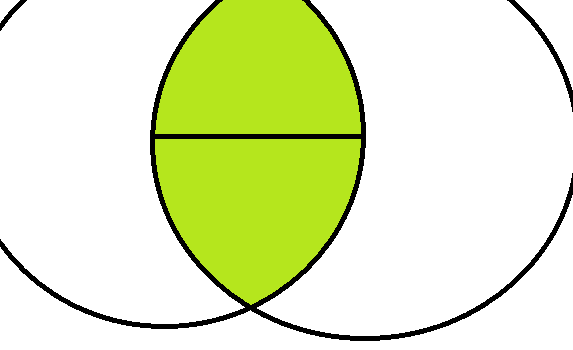# Trying to formulate a proof for this claim related to triangle inequality

(From outside the box geometry)

Claim: The longest side in a triangle must be opposite from the largest angle

Idea: Suppose there is some line L where L is the longest side for some triangle TThen, since neither of the remaining two sides of T can have a length greater than that of line L, the remaining one vertex of T, V, should be located among the area colored green.Clearly, the angle opposite to line L would be at its smallest when vertex V is located at the edge of the area colored green. Clearly, when vertex V is located at any point along the edge of the area colored green, the resulting triangle would either equilateral or bilateral, with the angle at vertex V always being either bigger than or equal to all other two angles.

Clearly, these pieces be enough to prove the original claim, if properly fitted and formulated together. However, making a formal proof is a process which I'm not very familiar with. Could someone perhaps help me with this?Note by 김 재형
3 years, 4 months ago

This discussion board is a place to discuss our Daily Challenges and the math and science related to those challenges. Explanations are more than just a solution — they should explain the steps and thinking strategies that you used to obtain the solution. Comments should further the discussion of math and science.

When posting on Brilliant:

• Use the emojis to react to an explanation, whether you're congratulating a job well done , or just really confused .
• Ask specific questions about the challenge or the steps in somebody's explanation. Well-posed questions can add a lot to the discussion, but posting "I don't understand!" doesn't help anyone.
• Try to contribute something new to the discussion, whether it is an extension, generalization or other idea related to the challenge.

MarkdownAppears as
*italics* or _italics_ italics
**bold** or __bold__ bold
- bulleted- list
• bulleted
• list
1. numbered2. list
1. numbered
2. list
Note: you must add a full line of space before and after lists for them to show up correctly
paragraph 1paragraph 2

paragraph 1

paragraph 2

[example link](https://brilliant.org)example link
> This is a quote
This is a quote
    # I indented these lines
# 4 spaces, and now they show
# up as a code block.

print "hello world"
# I indented these lines
# 4 spaces, and now they show
# up as a code block.

print "hello world"
MathAppears as
Remember to wrap math in $$ ... $$ or $ ... $ to ensure proper formatting.
2 \times 3 $2 \times 3$
2^{34} $2^{34}$
a_{i-1} $a_{i-1}$
\frac{2}{3} $\frac{2}{3}$
\sqrt{2} $\sqrt{2}$
\sum_{i=1}^3 $\sum_{i=1}^3$
\sin \theta $\sin \theta$
\boxed{123} $\boxed{123}$